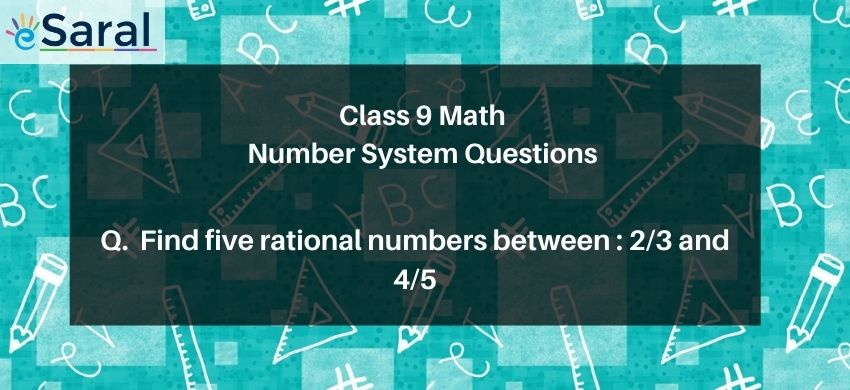Most Affordable JEE | NEET | 8,9,10 Preparation by Kota's Top IITian Doctor Faculties

# Find five rational numbers between:2/3 and 4/5# Question : State true or false:Five rational numbers between$\frac{2}{3}$ and $\frac{4}{5}$ are $\frac{41}{60}, \frac{42}{60}, \frac{43}{60}, \frac{44}{60}, \frac{45}{60}$

1. True
2. False
Solution :

Correct option is 1.True

To get the rational numbers between $\frac{2}{3}$ and $\frac{4}{5}$

Take an LCM of these two numbers: $\frac{10}{15}$ and $\frac{12}{15}$

Multiply numerator and denominator by $4: \frac{40}{60}$ and $\frac{48}{60}$

All the numbers between $\frac{40}{60}$ and $\frac{48}{60}$ form the answer

Some of these numbers are $\frac{41}{60}, \frac{42}{60}, \frac{43}{60}, \frac{44}{60}, \frac{45}{60}$

Hence the statement is true.